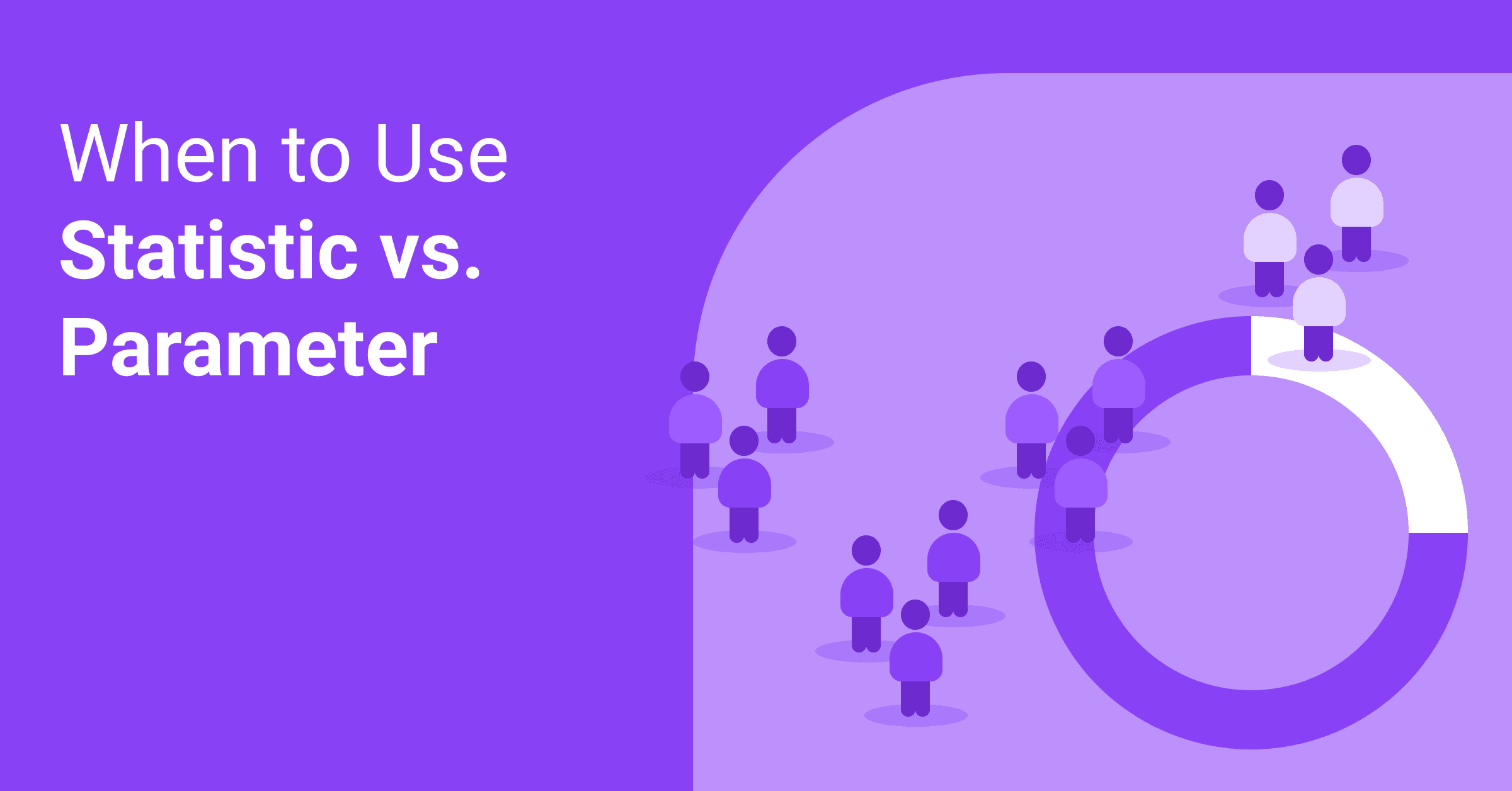KPIs & metrics

# Parameter vs Statistic – Definitions, Differences, Examples

Parameter and statistic are closely related terms that are important for the determination of the sample size. Many have trouble understanding the difference between parameter and statistic, but it’s important to know what exactly these measures mean and how to distinguish them.## Parameter vs. Statistic: A Definition

While a parameter is a fixed measure describing an entire population (a mass of all units under consideration that shares common characteristics) based on all the elements within that population, a statistic is a characteristic of a sample.

If you ask all employees in a factory what kind of lunch they prefer and half of them say pasta, you get a parameter here – 50% of the employees like pasta for lunch. On the other hand, it’s impossible to count how many men in the whole world like pasta for lunch, since you can’t ask all of them about their choice. In that case, you’d probably survey just a representative sample (a portion) of them and extrapolate the answer to the entire population of men. This brings us to the other measure called a statistic.

It’s a measure of characteristic saying something about a fraction (a sample) of the population under study. A sample in statistics is a part or portion of a population. The goal is to estimate a certain population parameter. You can draw multiple samples from a given population, and the statistic (the result) acquired from different samples will vary, depending on the samples. So, using data about a sample or portion allows you to estimate an entire population's characteristics.

## Parameter vs. Statistic: The Differences

The difference between a parameter vs a statistic is that a parameter is a fixed measure describing the whole population, while a statistic is a characteristic of a sample, a portion of the target population.

A parameter is a fixed, unknown numerical value, while the statistic is a known number and a variable which depends on the portion of the population.

Sample statistic and population parameters have different statistical notations:

In population parameter, population proportion is represented by P, mean is represented by µ (Greek letter mu), σ2 represents variance, N represents population size, σ (Greek letter sigma) represents standard deviation, σx̄ represents Standard error of the mean, σ/µ represents Coefficient of variation, (X-µ)/σ represents standardized variate (z), and σp represents standard error of proportion.

In sample statistics, mean is represented by x̄ (x-bar), sample proportion is represented by p̂ (p-hat), s represents standard deviation, s2 represents variance, the sample size is represented by n, sx̄ represents Standard error of the mean, sp represents standard error of a proportion, s/(x̄) represents Coefficient of variation, and (x-x̄)/s represents standardized variate (z).

### Examples of Parameters

• 20% of U.S. senators voted for a specific measure. Since there are only 100 senators, you can count what each of them voted.

### Examples of Statistic

• 50% of people living in the U.S. agree with the latest health care proposal. Researchers can’t ask hundreds of millions of people if they agree, so they take samples or part of the population and calculate the rest.## What Are The Differences Between Population Parameters and Sample Statistics?

The average weight of adult men in the U.S. is a parameter with an exact value – but we don’t know it.

Standard deviation and population mean are two common parameters.A statistic is a characteristic of a group of population or sample. You get sample statistics when you collect a sample and calculate the standard deviation and the mean. You can use sample statistics to make certain conclusions about an entire population, thanks to inferential statistics. But, it would help if you had particular sampling techniques to draw valid conclusions. Using these techniques ensures that samples deliver unbiased estimates – correct on average. When it comes to based estimates, they are systematically too low or too high, so you don’t need them.

To estimate population parameters in inferential statistics, you use sample statistics. For instance, if you collect a random sample of female teenagers in the U.S. and measure their weights, you can calculate the sample mean. You can use the sample mean as an unbiased estimate of the population mean.

## Is Standard Deviation a Statistic or Parameter?

It’s a statistic that measures the dispersion of the dataset relative to its mean. You can calculate the standard deviation as the square root of the variance. The variance, on the other hand, is the average of the squared differences from the mean.## Conclusion

Parameter vs. statistic – both are similar yet different measures. The first one describes the whole population, while the second describes a part of the population.

Published on Oct 09 2019WRITTEN BY

Indrė Jankutė-Carmaciu

Indrė is a copywriter at Whatagraph with extensive experience in search engine optimization and public relations. She holds a degree in International Relations, while her professional background includes different marketing and advertising niches. She manages to merge marketing strategy and public speaking while educating readers on how to automate their businesses.Whatagraph lets marketing agencies and in-house marketers create and send beautiful marketing reports in minutes instead of hours. Automate the way you collect, show and share marketing data with your clients and managers.